## Friday, 25 December 2009

### Solution to The Two Trains Puzzle

As promised I am going to post a solution to the two trains problem.
I restate the problem below:

Two trains are speeding towards each other on the same track both at a speed of 50 km/hr. At the instant they are 100 km apart Bumble Bee starts flying from the windscreen of the first train to the second train at a speed of 75 km/hr. When it reaches the windscreen of the second train it bounces back at the same speed to the other train. It continues repeating this cycle indefinitely. Determine the total. distance traveled by Bumble Bee until the instant it experiences an untimely death due to the catastrophic head on collision between these trains.

And now for the solution:

Here is a visualization of the problem:

And here is the solution:

First we take the instant the two trains are 100 km apart.
Bumble Bee will travel towards the second train and the second train will travel towards Bumble Bee for a time 't' until they meet. The distance travelled during this time must be 100 km.

75t +  50t = 100
125t = 100
t = 4/5

Now Bumble Bee travels towards the other train but this time the trains are only 20 km apart. So:

75t + 50t = 20
125t = 20
t = 4/25

Now Bumble Bee again travels towards the other train. But this time the trains are only 4 km apart. So the time until they meet will be 4/125.

What I am trying to do is to find the total time Bumble Bee spends bouncing between the trains. If I have that figure I can multiply it by the speed of Bumble Bee and get the distance. If you glance at the time you can immediately comprehend that the time keeps reducing but does not become zero unless I repeat the process of finding the time an infinite number of times. Serendipitously there exists a method of finding the sum of all those times that is better than spending an infinite amount of time adding an infinite amount of numbers.

We can express the total time T as an Infinite Geometric Series.

T = 4/5 + 4/25 + 4/125 + .................... ad infinitum
or

It takes only a moron to realize that this Infinite Geometric Series converges to 1. So the total amount of time Bumble Bee spends bouncing between the trains is 1 hour.

The total distance traveled is therefore 1 x 75 = 75 km
______
--------
And that is a truly beautiful result.

## Monday, 21 December 2009

### Winter Solstice

It is the day of the Winter solstice, the day on which the Earth is tilted as far away from the sun as possible. And so today at midday the sun would have been tilted away form normal by as much as 23.15 degrees at the equator..

Predictably the northern hemisphere will experience it's shortest duration of daylight today. Today was also the day I planned to recheck my calculation of the tilt of the Earth's axis. But alas! The weather is unaccommodating. Despite the fact that it was reasonably bright int he morning, just before noon, clouds started gathering ominously in the sky and any hopes of a measurement crashed.

## Thursday, 17 December 2009

### Another Calculation

Today I decided to do another calculation of interest related to the Earth.

I chose to calculate the speed of rotation of the Earth. I needed some reference point and therefore chose to calculate the speed of one point on the Equator. I considered  the day of the Equinox i.e the day on which the duration of daylight and night time would be exactly the same(12 hours). I imagined myself on a desert island in the sea which is located exactly on the equator. On the day of the Equinox the sun would travel 180 degrees from the west to the east. From this assumption I obtained a crucial figure: The angular velocity of the Earth. Since 180 degrees in pi radians the angular velocity would be pi/12 radians per hour.
The radius of the earth is 6367.5 km. The velocity of one point on the Equator would therefore be (pi/12) * (6367.5) = 1674.365 km/hr or 463.05766716974556587756670701464 m/s.

But that was easy I did not even have to make any physical measurements. I decided to make a very interesting calculation: The difference between the speed of my feet and the speed of the top of my head. I state the radius of the Earth in meters: 6367500 m. my height is 1.67 m.
The speed of the top of my head is.  463.05778861......(I am not displaying all 32 digits in my calculator display but I use all the digits for my calculation.)
Subtracting the speeds I find that my head is 0.0001214458271179387663942735177012 m/s faster than my foot. So during the day my foot only travels   40008182.443466016891821763486064 m while my head travels 40008192.936385479881731179951293 m. Subtracting, I get that my head travels 10.5 meters more than my foot in one day!!

Now I apply Einstein's Relativistic equation for time dilation:

The relative speed between my head and  my foot or v is 0.0001214458271179387663942735177012 m/s.
c is the speed of light. Let t-zero be 1.
I get t-v to be  1.0000000000000000000000000820529 seconds
This means that for every second that passes for my head 1.0000000000000000000000000820529 seconds pass for my foot.

The average life expectancy of the world is 65 years. If I live to be 65, my head will have lived a total of 2049840000 seconds while my foot will have lived a total of 2049840000.000000000000000168195316536 seconds.
This means that at the end of 65 years my foot will be older than my head by 1.7 * 10^-16 or

0.000000000000000168195316536

seconds!!

I think you would agree that this is a truly astounding revelation.




## Wednesday, 16 December 2009

### A Delightful Little Problem

Here is a tricky little puzzle:

Two trains are speeding towards each other on the same track both at a speed of 50 km/hr. At the instant they are 100 km apart Bumble Bee starts flying from the windscreen of the first train to the second train at a speed of 75 km/hr. When it reaches the windscreen of the second train it bounces back at the same speed to the other train. It continues repeating this cycle indefinitely. Determine the total. distance traveled by Bumble Bee until the instant it experiences an untimely death due to the catastrophic head on collision between these trains.

It shouldn't take more than five minutes and requires only a basic understanding of mathematics and a bit of logical reasoning. I invite the readers to try to solve this puzzle and post your solutions in the comments below.(A word of advice: Make sure YOU do the problem and please try to do yourself justice by abstaining from searching for a solution online.) I will be posting a solution to this problem in one of the following posts.

## Sunday, 13 December 2009

### A Few Clarifications.

In my entry before the last there was a lot going on behind the scenes which I didn't explain. For the benefit of my scientifically inclined readers I am going to explain the the chain of reasoning I went through to arrive at the final answer and why my answer was as accurate as it was. One of my friends had sent me an email with an inquiry of  how I reasoned it out. Here I present my reply. I hope it clears all doubts.

To do this calculation I had to make an assumption.

First of all, I had to assume that the earth is tilted as far as possible away from the sun. Since It is December Which is winter time in the northern hemisphere, this is a pretty good approximation. But I couldn't be sure. However something else partially cancels this effect(namely, the latitude of my position).

I reasoned that since it was only the 8th of December, the earth could not have reached this maximum position(In actual fact, maximum is reached on December 22). So the angle that I calculate must be smaller than 23.45 degrees. I the checked the location of the city I stay in and I found out that it was on the 5 degree North latitude. So I reasoned that even if the angle would be smaller this smaller angle I get would be larger than the original tilt by five degrees (Since I'm 5 degrees above the equator). So if the earth was not at this maximum position, my angle would be made larger by roughly 5 degrees and my answer would therefore be closer to the correct answer.

And most importantly I made this calculation at exactly 12 noon, a time when the sun should have been directly above my head making an angle of 90 degrees. If I made the calculation at this time, then any deviation suffered by the sun from normal must be the tilt of the earth.

So I have taken into account the Latitude (5 degrees greater), and the time of the day. I do not need to worry about the longitude because all places on the same longitude will experience midday at exactly the same time. I think that covers everything.

## Friday, 11 December 2009

### If Zero by Zero were defined....

If zero by zero were defined a whole lot of crazy things would start to happen.
Just as an example I quote this section of Wikipedia.

With the following assumptions: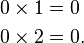\begin{align} 0\times 1 &= 0 \\ 0\times 2 &= 0. \end{align}
The following must be true: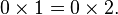$0\times 1 = 0\times 2.\,$
Dividing by zero gives: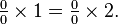$\textstyle \frac{0}{0}\times 1 = \frac{0}{0}\times 2.$
Simplified, yields: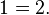$1 = 2.\,$
The fallacy is the implicit assumption that dividing by 0 is a legitimate operation with 0/0 = 1.
Although most people would probably recognize the above "proof" as fallacious, the same argument can be presented in a way that makes it harder to spot the error. For example, consider the following equations: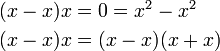\begin{align} (x-x)x &= 0 = x^2-x^2 \\ (x-x)x &= (x-x)(x+x) \end{align}
Dividing by x − x gives: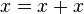$x = x+x\,$
and dividing by x gives:$1 = 2.\,$
Therefore it would  be possible to prove that 1 = 2 and 2=3. In fact it would be within our capability to prove that any number in the number system is equal to any other number. If this were true then everything would be exactly the the same.It is therefore obvious that division by zero is undefined. If zero by zero were defined all the buildings and bridges would tumble and crumble. All calculations would be rendered useless. You wouldn't be able to tell the difference between an elephant and an ant. If zero by zero were defined, there would be no difference between you and me, no difference between big and small, no difference between hot and cold. The universe would be astoundingly uniform. There would be no stars or galaxies or beautiful nebulae. No black holes, no Quasars, no interstellar dust. The universe would be just one big boring entity.

## Tuesday, 8 December 2009

### The Tilt of the Earth's Axis

We are all aware of the fact that the Earth's axis is tilted from the normal. This tilt is the cause of the seasons.  Today , to relieve myself of boredom I decided to calculate this tilt of the Earth's axis using a very tall lamp post and its shadow both of which could be observed from my bedroom window.
The Tilt
I started with the shadow. I measured the length of the shadow using my normal 6.5 inch (15 cm) ruler. It recorded a length of 3.5 cm. Careful to hold my head so that it's spatial co-ordinates remain reasonably constant I measured the height of the lamp post. I knew that all angles on the triangle I constructed(See illustration below) would be exactly the same as those in the triangle formed by the original lamp post and shadow because I was working with similar triangles.

So I did the math.
Theta would be the arctan of (8/3.5).
θ = 66.4°

The angle of tilt (φ):
φ = 90 - 66.4°
φ = 23.6°
________
-----------

The actual angle of tilt of the Earth's axis is 23.45°. The error in my calculation is therefore 0.6% which is absolutely fantastic.

And that is the story of how I measured the angle at which the Earth's axis tilts away from the normal.